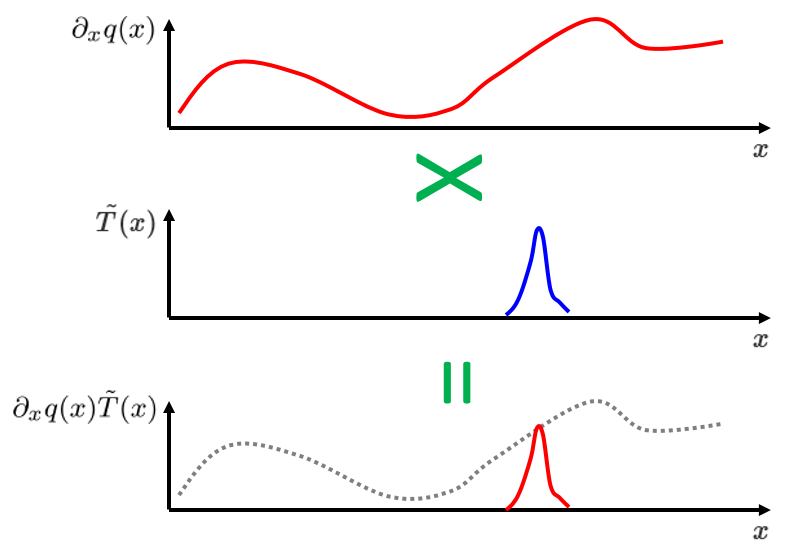# 弱形式概述

2014年 11月 19日

### 一个简单示例

(1)

q(x)=-\partial_x T(x)

(2)

\partial_x q(x)=0

1. 我们希望专注于弱形式的核心思想，不希望因复杂物理系统中的数学而分散注意力。
2. 后续博客中，我们会拓展到涉及多个域的系统，以便阐述两个方程组在边界条件处的耦合。如果现在就开始研究复杂的案例，那等到拓展案例时主题将会变得非常晦涩难懂。

### 弱公式化

\int_1^5 \partial_x q(x) \,dx = 0

\int_{3.49}^{3.51} \partial_x q(x) \,dx = 0

(3)

\int_1^{1.01} \partial_x q(x) \,dx = 0 \mbox{ , }\int_{1.01}^{1.02} \partial_x q(x) \,dx = 0 \mbox{ , }\int_{1.02}^{1.03} \partial_x q(x) \,dx = 0 \mbox{ , . . . }(4)

\int_1^5 \partial_x q(x) \tilde{T}(x) \,dx = 0

### 降低求导阶数

(5)

q(x=5) \tilde{T}(x=5)-q(x=1) \tilde{T}(x=1)-\int_1^5 q(x) \partial_x \tilde{T}(x) \,dx = 0

### 自然边界条件

(6)

\int_1^5 \partial_x T(x) \partial_x \tilde{T}(x) \,dx = -\Lambda_1 \tilde{T}_1 -\Lambda_2 \tilde{T}_2

### 固定边界条件

(7)

\int_1^5 \partial_x T(x) \partial_x \tilde{T}(x) \,dx = -2 \tilde{T}_1 -\lambda_2 \tilde{T}_2 -\tilde{\lambda}_2 (T_2-9)

### 总结及下一篇博客

#### 评论 (8)

##### 留言##### 峰 程
2016-04-06

Dear Chien,
Thanks for your article. I have some questions about it.
First, I can’t understand the derivation of Eq.(4). I think Eq.(4) should be written as
[int_1^5 {{partial _x}qleft( x right)delta left( {x – y} right)} dx = 0]
with
[1 le y le 5].
Here I used the Dirac delta function to replace the test function. It can be replaced by other “narrow” functions, but its strictness will be “weakened”.
Can you tell me a little more about the test function so I can relate it with the Dirac delta function?
Many thanks.
Sincerely,
Feng##### Chien Liu
2016-04-07

Dear Feng,

It will become clear later in this blog:
https://www.comsol.com/blogs/discretizing-the-weak-form-equations/
why the Dirac delta function would not be a good choice as the test function for numerical analysis. Roughly speaking we need the basis function to have some finite width in order to reconstruct the solution function. For more in-depth discussion on this topic, you may want to take a look at the references listed in the comments of this blog:
https://www.comsol.com/blogs/implementing-the-weak-form-in-comsol-multiphysics/

Sincerely,

Chien##### 峰 程
2016-04-08##### Chien Liu
2016-04-12

Dear 峰 程,
You can think of lambda2 as the heat flux needed to keep the boundary temperature T2 at the specified value of 9. So here we are instructing the software to “adjust the value of the heat flux lambda2 at the boundary, until the boundary temperature T2 reaches the value of 9”. Does this make sense?
Sincerely,
Chien##### zidong song
2017-07-19##### 宇航 秦
2017-07-21

Song Zidong, 您好！

Email: support@comsol.com##### 梓鹏 梁
2018-07-26##### Chien Liu
2018-07-26

Dear 梓鹏, Thank you for the question. One way to think about this is to consider a problem of constrained optimization. Then the last two terms in equation 7 come from taking the variation operation on the product of the Lagrange multiplier (labmda_2) and the constraint (T_2-9). Sincerely, Chien